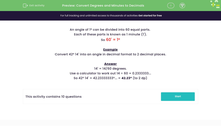# Convert Degrees and Minutes to Decimals

In this worksheet, students will convert angles given in degrees and minutes to angles in decimal format.Key stage:  KS 3

Curriculum topic:   Number

Curriculum subtopic:   Use Standard Units of Measure

Popular topics:   Angles worksheets

Difficulty level:#### Worksheet Overview

An angle of 1º can be divided into 60 equal parts.

Each of these parts is known as 1 minute (1').

So 60' = 1º

Example

Convert 42º 14' into an angle in decimal format to two decimal places.

14' = 14/60 degrees.

Use a calculator to work out 14 ÷ 60 = 0.2333333...

So 42º 14' = 42.23333333º... = 42.23º (to 2 dp)

Let's try some questions.

### What is EdPlace?

We're your National Curriculum aligned online education content provider helping each child succeed in English, maths and science from year 1 to GCSE. With an EdPlace account you’ll be able to track and measure progress, helping each child achieve their best. We build confidence and attainment by personalising each child’s learning at a level that suits them.

Get started